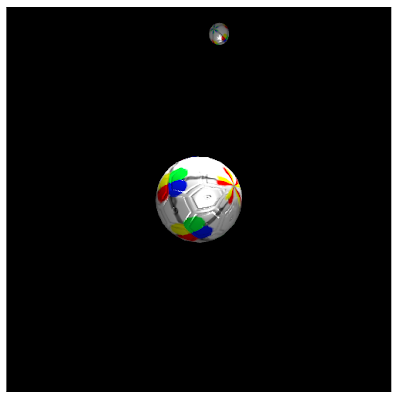## Sunday, June 17, 2012

### Rotation in CubicVR.js

‹prev | My Chain | next›

I have learned quite a bit about Gladius and CubicVR.js over the past several days. There is still much that eludes me. Among the myriad of gaming and rendering tidbits that continues to baffle me is how things move.

Actually, I know how the objects rotate in the sample that I continue to evolve from one of the examples bundled with Gladius:Both objects in that scene rotate. The large object in the center rotates in all directions, though it stays in the center of the scene. The smaller object rotates as it revolves about the central object—almost like a moon about a planet (except that this moon is also a light source shining on the planet).

As I mentioned, I know how the rotation works. First, the example grabs the rotation transform property from the object and casts it into a three dimensional vector object:
`var cubeRotation = new engine.math.Vector3( space.findNamed( "cube" ).findComponent( "Transform" ).rotation );`
(the "cube" in the variable name is an artifact of the original example)

Given the current angles of rotation, we add a little bit of rotation in the X direction, a little less in the Y direction, and a little more in the Z direction:
`cubeRotation = engine.math.vector3.add( cubeRotation, [space.clock.delta * 0.003, space.clock.delta * 0.001, space.clock.delta * 0.0007] );`
In the above, the `space.clock.delta` is the amount of time that has passed since the current animation task was last run. Even if the task is skipped for a cycle or two, the rate of rotation will remain fixed by virtue of moving a the same rate over time.

Lastly, the new rotation is applied to the object:
`space.findNamed( "cube" ).findComponent( "Transform" ).setRotation( cubeRotation );`
And it works. I can vary the components of the rotation vector and observe changes in how both objects rotate.

So I understand rotation, but I do not know how the "moon" revolves about the "planet". There is no corresponding `setRevolution()` method call for the moon. In every way that I can see, the movement of the moon / light source is nothing more than a simple rotation:
``````      var lightRotation = new engine.math.Vector3( space.findNamed( "light-center" ).findComponent( "Transform" ).rotation );
lightRotation = engine.math.vector3.add( lightRotation, [0, space.clock.delta * 0.001, 0] );
space.findNamed( "light-center" ).findComponent( "Transform" ).setRotation( lightRotation );``````
There are no other `task()` functions assigned to the Gladius engine. There is no mention of motion at all as far as I can tell. So...

My first inclination is that this is somehow a property of the light source. There is nothing that jumps out at me about the light source's definition:
``````    var lightDefinition = new cubicvr.LightDefinition({
intensity: 2,
light_type: cubicvr.LightDefinition.LightTypes.POINT,
method: cubicvr.LightDefinition.LightingMethods.DYNAMIC
})
// ...
[
new engine.core.Transform( [3, 0, 0], [0, 0, 0], [1, 1, 1] ),
new cubicvr.Light( lightDefinition )
]
));``````
With nothing else to try, I mucking with the rotation. Instead of rotating about the Y-axis (up and down), I try rotation about the Z-axis:
``      lightRotation = engine.math.vector3.add( lightRotation, [0, 0, space.clock.delta * 0.001] );``
The result is that the "moon" / light source is now revolving up and down (around the Z-axis):Ah. I had mistakenly assumed that moving the moon also created a new frame of reference about which the rotation took place. It seems that, although motion of objects is independent of each other, the frame of reference is not.

That actually clears up quite a bit for me.

Day #420

1.This part of the example could use some comments, but I'll explain what's happening. The orbiting box is done with a bit of a trick. Rather than compute its orbit manually, I created a second object, light-center, and set that as the parent for light-source. The task rotates light-center, which in turn causes light-source to revolve around it. This happens because of the parent-child relationship between the objects. In the example, light-center has only a transform so it isn't visible in the simulation.

1.Ah, thanks. I had suspected that might be the case, but changing the rotation properties on task seemed to suggest that rotation was independent of the light-center / parent-child stuff. I'll explore a bit more tonight.

2.Hey Chris, may you post your source for this orbiting example. It'd be most helpful. Thanks.

1.It's been a while, but I believe that the source code for this post ended up in: https://github.com/eee-c/gladius-my/blob/master/public/sphere.html